GFG App
Open AppBrowser
Continue

# Class 9 NCERT Solutions- Chapter 1 Number System – Exercise 1.3

### (i) 36/100

Solution:

In the given question, we get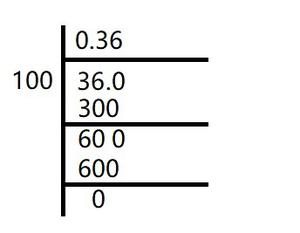Here, the remainder becomes zero.

Hence, decimal expansion becomes terminating.

36/100 = 0.36

### (ii) 1/11

Solution:

In the given question, we get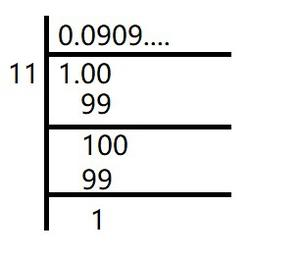Here, the remainder never becomes zero and remainders repeat after a certain stage.

Hence, decimal expansion is non-terminating recurring

1/11 =### (iii)Solution:

Here,In the given question, we get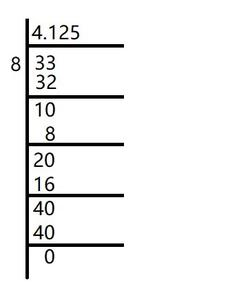Here, the remainder becomes zero.

Hence, decimal expansion becomes terminating.= 4.125

### (iv) 3/13

Solution:

In the given question, we get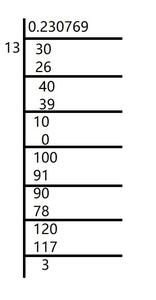Here, the remainder never becomes zero and remainders repeat after a certain stage.

Hence, decimal expansion is non-terminating recurring

3/13 =### (v) 2/11

Solution:

In the given question, we get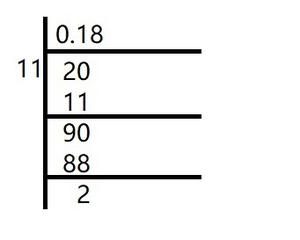Here, the remainder never becomes zero and remainders repeat after a certain stage.

Hence, decimal expansion is non-terminating recurring

2/11 =### (vi) 329/400

Solution:

In the given question, we get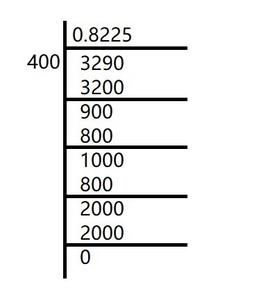Here, the remainder becomes zero.

Hence, decimal expansion becomes terminating.

329/400 = 0.8225

### [Hint: Study the remainders while finding the value of 1/7 carefully.]

Solution:

As it is given,So,### (i)Solution:= 0.66666……

Lets’s take, x = 0.66666……

10x = 6.666….

So,

10x – x = (6.6666…..) – (0.66666……..)

9x = 6

x = 6/9

x = 2/3

Hence, x is in the form p/q, here p and q are integers and q ≠ 0.

### (ii)Solution:= 0.4777777……

Lets’s take, x = 0.4777777……

10x = 4.77777…….

So,

10x – x = (4.77777…….) – (0.4777777……)

9x = 4.3

9x = 43/10

x = 43/90

Hence, x is in the form p/q, here p and q are integers and q ≠ 0.

### (iii)Solution:= 0.001001001……

Lets’s take, x = 0.001001001……

1000x = 1.001001001……

So,

1000x – x = (1.001001001……) – (0.001001001……)

999x = 1

x = 1/999

Hence, x is in the form p/q, here p and q are integers and q ≠ 0.

### Question 4. Express 0.99999 …. in the form p/q, Are you surprised by your answer? With your teacher and classmates discuss why the answer makes sense.

Solution:

Lets’s take, x = 0.99999……

10x = 9.99999….

So,

10x – x = (9.99999…..) – (0.99999……..)

9x = 9

x = 1

As, 0.9999….. just goes on, then at some point of time there is no gap between 1 and 0.9999….

We can observe that, 0.999 is too much near 1, hence, 1 is justified as the answer.

Hence, x is in the form p/q, where p and q are integers and q ≠ 0.

### Question 5. What can the maximum number of digits be in the repeating block of digits in the decimal expansion of 1/17? Perform the division to check your answer.

Solution:

In the given question,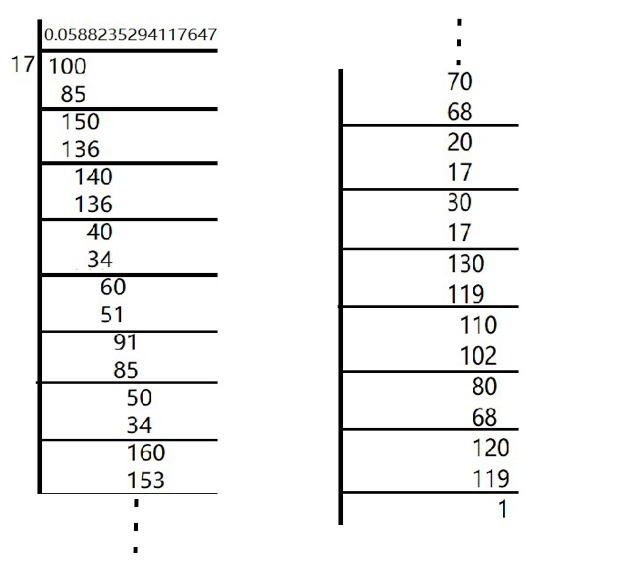There are 16 digits in the repeating block of the decimal expansion of 1/17

Here, the remainder never becomes zero and remainders repeat after a certain stage.

Hence, decimal expansion is non-terminating recurring

1/17 =### Question 6. Look at several examples of rational numbers in the form p/q (q ≠ 0), where p and q are integers with no common factors other than 1 and having terminating decimal representations (expansions). Can you guess what property q must satisfy?

Solution:

We observe that when q is 2, 4, 5, 8, 10… Then the decimal expansion is terminating.

Let’s take some example,

1/2 = 0. 5, denominator q = 21

7/8 = 0. 875, denominator q =23

4/5 = 0. 8, denominator q = 51

So, we conclude that terminating decimal may be obtained in the situation where

prime factorization of the denominator of the given fractions has the power

of only 2 or only 5 or both.

In the form of 2m × 5n, where n, m are natural numbers.

### Question 7. Write three numbers whose decimal expansions are non-terminating non-recurring.

Solution:

As we know that all irrational numbers are non-terminating non-recurring.

So,

√5 = 2.23606798…….

√27 =5.19615242……

√41 = 6.40312424…..

### Question 8. Find three different irrational numbers between the rational numbers 5/7 and 9/11.

Solution:

As, decimal expansion of

5/7 =9/11 =Hence, three different irrational numbers between them can be as follows,

0.73073007300073000073…

0.75075007300075000075…

0.76076007600076000076…

### (i) √23

Solution:

√23 = 4.79583152……

As the number is non-terminating non-recurring.

It is an irrational number.

### (ii) √225

Solution:

√225 = 15 = 15/1

As the number can be represented in p/q form, where q ≠ 0.

It is a rational number.

### (iii) 0.3796

Solution:

As, the number 0.3796, is terminating.

It is a rational number.

### (iv) 7.478478…

Solution:

As, the number 7.478478, is non-terminating but recurring.

It is a rational number.

### (v) 1.101001000100001…

Solution:

As, the number 1.101001000100001…, is non-terminating but recurring.

It is a rational number.

My Personal Notes arrow_drop_up